# sklearn.metrics.accuracy_score¶

sklearn.metrics.accuracy_score(y_true, y_pred, *, normalize=True, sample_weight=None)[source]

Accuracy classification score.

In multilabel classification, this function computes subset accuracy: the set of labels predicted for a sample must exactly match the corresponding set of labels in y_true.

Read more in the User Guide.

Parameters:
y_true1d array-like, or label indicator array / sparse matrix

Ground truth (correct) labels.

y_pred1d array-like, or label indicator array / sparse matrix

Predicted labels, as returned by a classifier.

normalizebool, default=True

If False, return the number of correctly classified samples. Otherwise, return the fraction of correctly classified samples.

sample_weightarray-like of shape (n_samples,), default=None

Sample weights.

Returns:
scorefloat

If normalize == True, return the fraction of correctly classified samples (float), else returns the number of correctly classified samples (int).

The best performance is 1 with normalize == True and the number of samples with normalize == False.

balanced_accuracy_score

Compute the balanced accuracy to deal with imbalanced datasets.

jaccard_score

Compute the Jaccard similarity coefficient score.

hamming_loss

Compute the average Hamming loss or Hamming distance between two sets of samples.

zero_one_loss

Compute the Zero-one classification loss. By default, the function will return the percentage of imperfectly predicted subsets.

Notes

In binary classification, this function is equal to the jaccard_score function.

Examples

>>> from sklearn.metrics import accuracy_score
>>> y_pred = [0, 2, 1, 3]
>>> y_true = [0, 1, 2, 3]
>>> accuracy_score(y_true, y_pred)
0.5
>>> accuracy_score(y_true, y_pred, normalize=False)
2


In the multilabel case with binary label indicators:

>>> import numpy as np
>>> accuracy_score(np.array([[0, 1], [1, 1]]), np.ones((2, 2)))
0.5


## Examples using sklearn.metrics.accuracy_score¶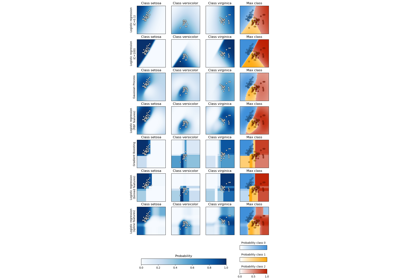Plot classification probability

Plot classification probability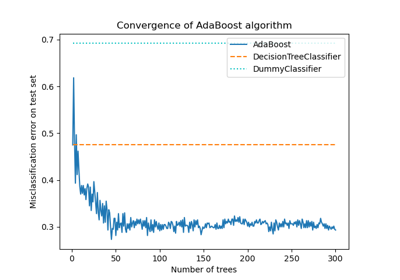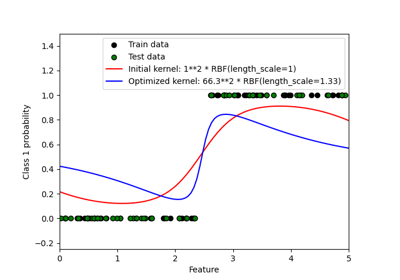Probabilistic predictions with Gaussian process classification (GPC)

Probabilistic predictions with Gaussian process classification (GPC)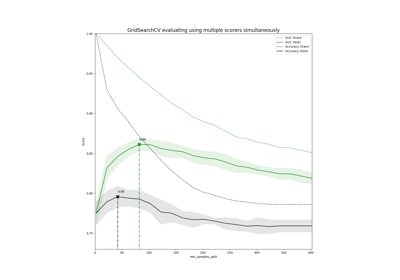Demonstration of multi-metric evaluation on cross_val_score and GridSearchCV

Demonstration of multi-metric evaluation on cross_val_score and GridSearchCV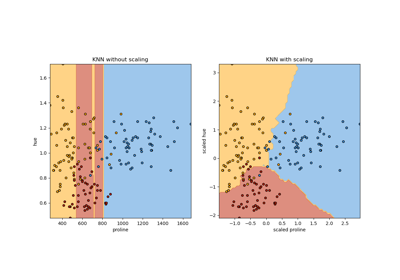Importance of Feature Scaling

Importance of Feature Scaling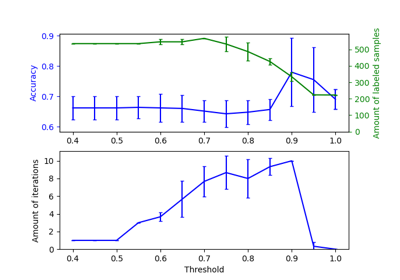Effect of varying threshold for self-training

Effect of varying threshold for self-training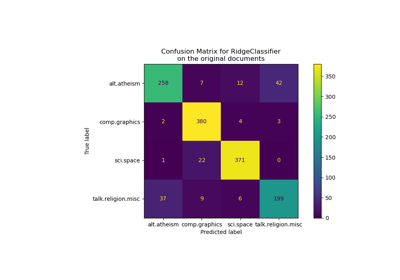Classification of text documents using sparse features

Classification of text documents using sparse features